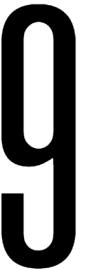Language: English | España | РусскийShare image:

Keywords: Number 9 PNG size: 92x270px, 9 PNG images, PNG image: Number 9 PNG, free PNG image, 9
Image category: 9
Format: PNG image with alpha (transparent)
Resolution: 92x270
Size: 4 kb

# Number 9 PNG image with transparent background | number9_PNG19111.png

This image has format transparent PNG with resolution 92x270.

# 9

9 (nine) is the natural number following 8 and preceding 10.

9 is a composite number, its proper divisors being 1 and 3. It is 3 times 3 and hence the third square number. Nine is a Motzkin number. It is the first composite lucky number, along with the first composite odd number and only single-digit composite odd number.

9 is the only positive perfect power that is one more than another positive perfect power, by Mihăilescu's Theorem.

9 is the highest single-digit number in the decimal system. It is the second non-unitary square prime of the form (p2) and the first that is odd. All subsequent squares of this form are odd.

Since 9 = 321, 9 is an exponential factorial.

A polygon with nine sides is called a nonagon or enneagon. A group of nine of anything is called an ennead.